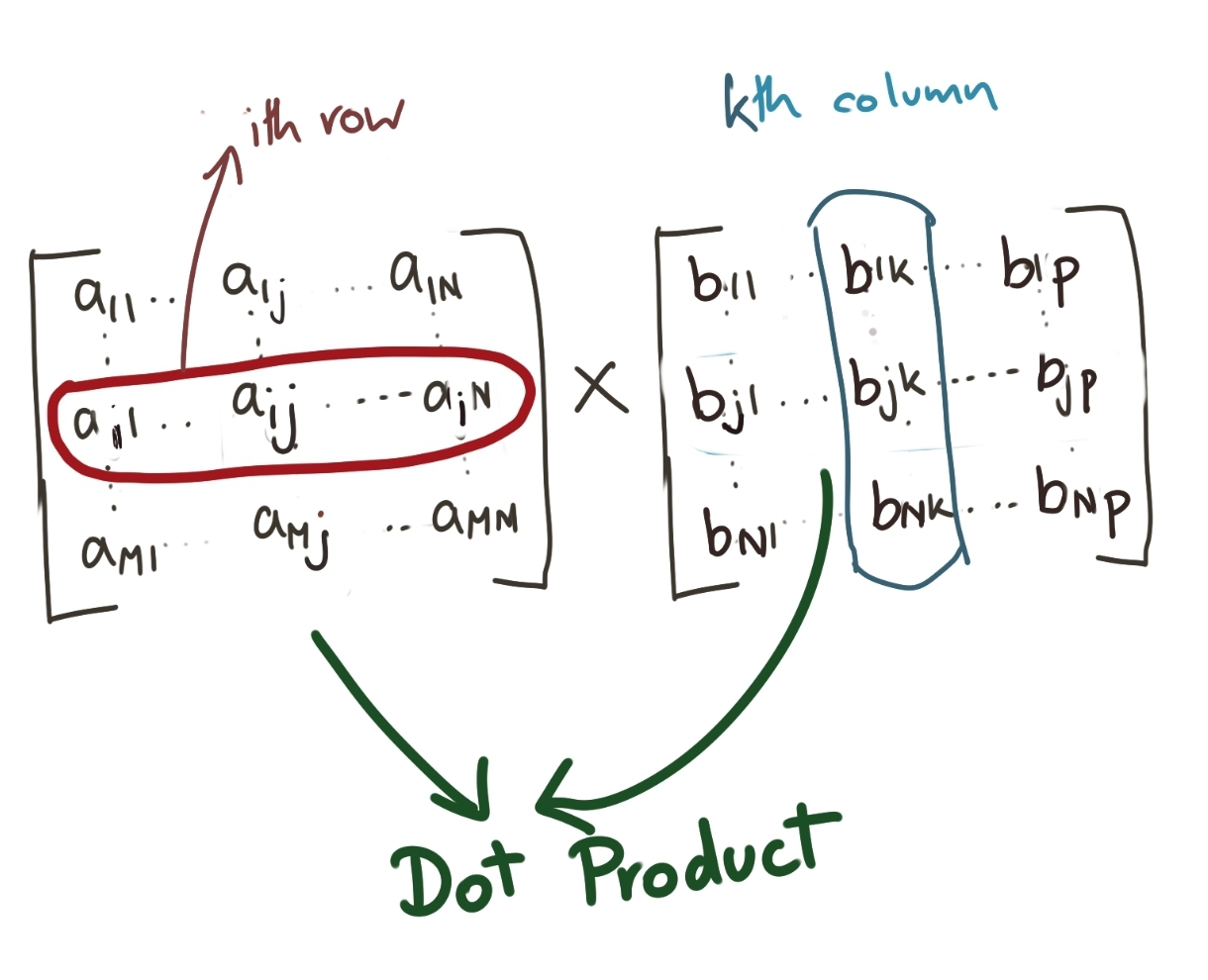# Total Internal Reflection

Technology and Art

# Matrix Outer Product: Value-wise computation and the Transposition Rule

Avishek Sen Gupta on 1 April 2021

We will discuss the value-wise computation technique for matrix outer products. This will lead us to a simple sketch of the proof of reversal of order for transposed outer products.

## Value-wise Computation

This is probably the method most widely used in high school algebra. You are essentially viewing this as a value-by-value computationThere is a very simple mnemonic for remembering it, namely:

The element $$Y_{ik}$$ in the $$i$$th row and the $$k$$th column is the dot product of the $$i$$th row vector (of the Left Hand Matrix) amd the $$k$$th column vector (of the Right Hand Matrix).This is also something which makes it obvious that the number of columns of the left matrix should be equal to the number of rows of the right matrix, because the dot product involves pairwise multiplication, and that cannot happen if the number of components in the row vector and the column vector are unequal.

Mathematically, each element is computed as below:

$Y_{ik}=A_{i1}B_{1j}+A_{i2}B_{2j}+ ... +A_{ij}B_{jk}+...+A_{iN}B_{Nj}$

or, more compactly:

$Y_{ik}=\displaystyle \sum_{j=1}^{j} A_{ij}B_{jk}$

To reiterate, it is important for the number of columns of $$A$$ and the number of rows $$B$$ to be equal for matrix multiplication to be a valid operation. In this case, this number is $$N$$. This important for proving why the order itself needs reversing, as you will see.

### Proof of Order Reversal in Transpose of an Operation

Assume we have two matrices, $$A$$ ($$M\times N$$) and $$B$$ ($$N\times P$$), which we multiply to get $$Y$$, i.e.,

$Y=AB$

We’d like to know what $$Y^T$$ looks like, in terms of $$A^T$$ and $$B^T$$. I’ll attempt to elaborate the thought process while writing the identities.

From the definition of transpose, we know that $${Y_{ik}}^T=Y_{ki}$$. Also, by definition of the value-wise computation of two matrices A and B, we have:

$Y_{ik}=\displaystyle \sum_{j=1}^{N} A_{ij}B_{jk}$

Now, I’d like to express $${Y_{ik}}^T$$ in terms of $$A^T$$ and $$B^T$$. Now, in the transpose versions of A and B, where have the $$i$$th row of A and the $$k$$th column of B moved to?

$$i$$th row of A has become the $$i$$th column of $$A^T$$ $$k$$th column of B has become the $$k$$th row of $$B^T$$

Mathematically, this is expressed as: $${A^T}_{ij}=A_{ji} \\ {B^T}_{jk}=B_{kj}$$

In order to preserve the property $${Y_{ik}}^T=Y_{ki}$$, we want the dot product of the $$i$$th column of $$A^T$$ (which is the $$i$$th row of $$A$$) with the $$k$$th row of $$B^T$$ (which is the $$k$$th column of $$B$$)

$Y_{ki}=\displaystyle \sum_{j=1}^{N} A_{ji}B_{kj} \\ Y_{ki}=\displaystyle \sum_{j=1}^{N} B_{kj}A_{ji} \\ Y_{ki}=\displaystyle \sum_{j=1}^{N} {B^T}_{jk}{A^T}_{ij}$

This is the value-wise computation of two matrices $$B^T$$ and $$A^T$$. Thus:

$(AB)^T=B^TA^T$

Note the important reversal of $$A_{ji}B_{kj}$$ to $$B_{kj}A_{ji}$$. The result is the same since both of $$A_{ji}$$ and $$B_{kj}$$ are simple scalars. However, matrix multiplication can only succeed if the columns of the left matrix and rows of the right matrix are equal (which is why we iterate over j from 1 to $$N$$; see the dimensions of the matrices $$A$$ and $$B$$).

## Conclusion

This rule of swapping the order of the outer product will also apply to when we are calculating inverses.

tags: Machine Learning - Linear Algebra - Theory